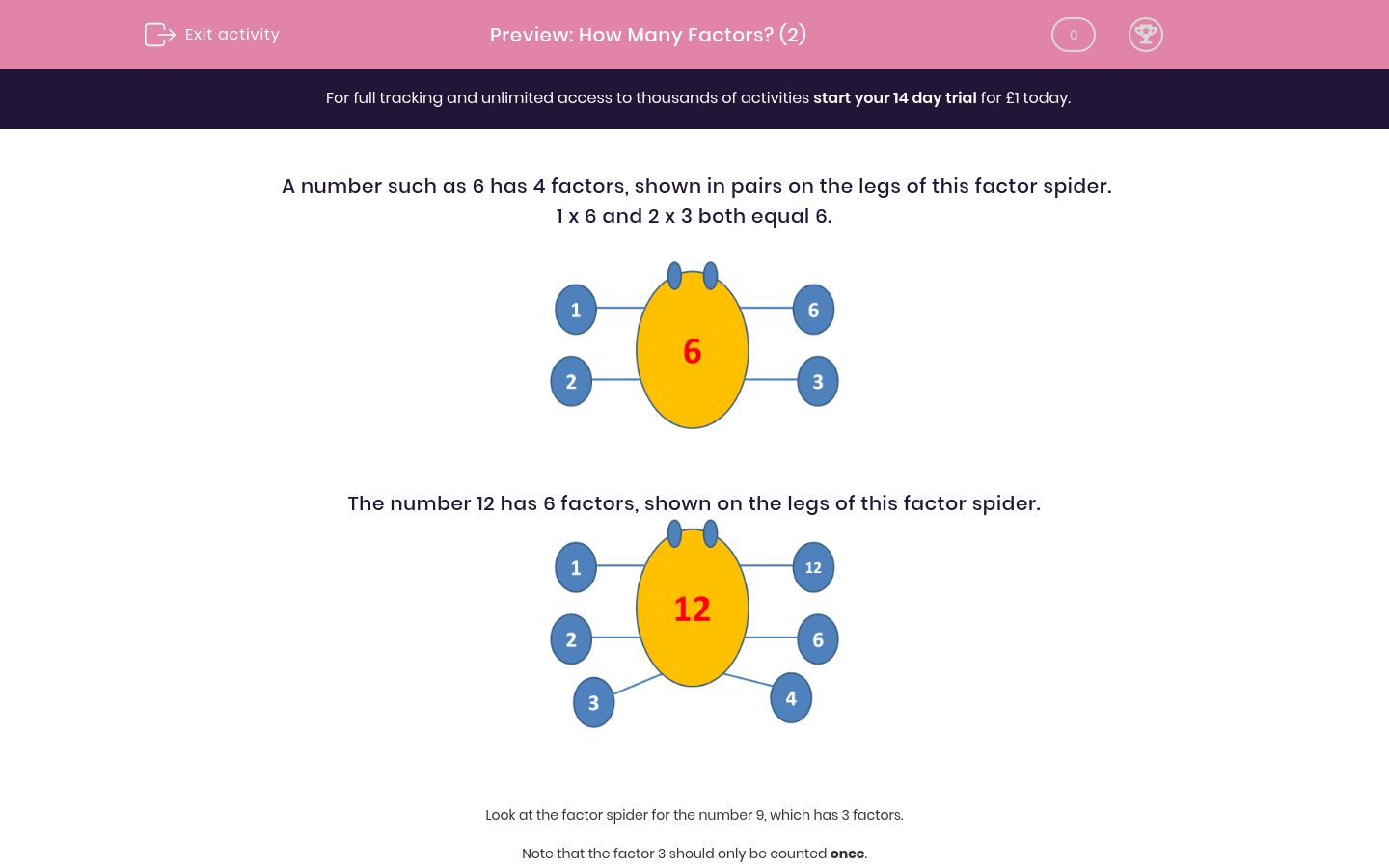# How Many Factors? (2)

In this worksheet, students state how many factors a number has by looking at its "factor spider"!Key stage:  KS 2

Curriculum topic:   Maths and Numerical Reasoning

Curriculum subtopic:   Factors and Multiples

Difficulty level:### QUESTION 1 of 10

A number such as 6 has 4 factors, shown in pairs on the legs of this factor spider.

1 x 6 and 2 x 3 both equal 6.The number 12 has 6 factors, shown on the legs of this factor spider.Look at the factor spider for the number 9, which has 3 factors.

Note that the factor 3 should only be counted once.How many factors does the following number have?

10

How many factors does the following number have?

15

How many factors does the following number have?

18

How many factors does the following number have?

13

How many factors does the following number have?

21

How many factors does the following number have?

40

How many factors does the following number have?

41

How many factors does the following number have?

35

How many factors does the following number have?

37

How many factors does the following number have?

36

• Question 1

How many factors does the following number have?

10

4
• Question 2

How many factors does the following number have?

15

4
• Question 3

How many factors does the following number have?

18

6
• Question 4

How many factors does the following number have?

13

2
• Question 5

How many factors does the following number have?

21

4
• Question 6

How many factors does the following number have?

40

8
• Question 7

How many factors does the following number have?

41

2
• Question 8

How many factors does the following number have?

35

4
• Question 9

How many factors does the following number have?

37

2
• Question 10

How many factors does the following number have?

36

9
---- OR ----

Sign up for a £1 trial so you can track and measure your child's progress on this activity.

### What is EdPlace?

We're your National Curriculum aligned online education content provider helping each child succeed in English, maths and science from year 1 to GCSE. With an EdPlace account you’ll be able to track and measure progress, helping each child achieve their best. We build confidence and attainment by personalising each child’s learning at a level that suits them.

Get started